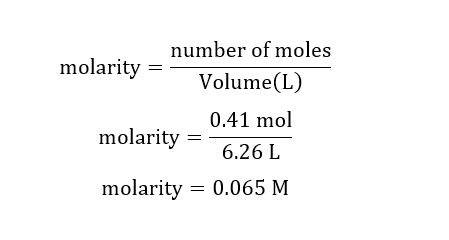# Calculate the molarity of the following solution0.41 mol of LiNO3 in 6.26 L of solution

Question
35 views

Calculate the molarity of the following solution

0.41 mol of LiNO3 in 6.26 L of solution

check_circle

Step 1

Given:

Volume of solution = 6.26 L

Moles of LiNO3 = 0.41 mol

Step 2

Calculation for molarity of LiNO3 solution:...

### Want to see the full answer?

See Solution

#### Want to see this answer and more?

Solutions are written by subject experts who are available 24/7. Questions are typically answered within 1 hour.*

See Solution
*Response times may vary by subject and question.
Tagged in